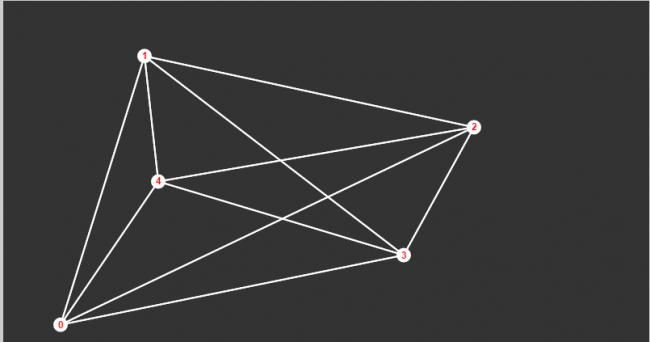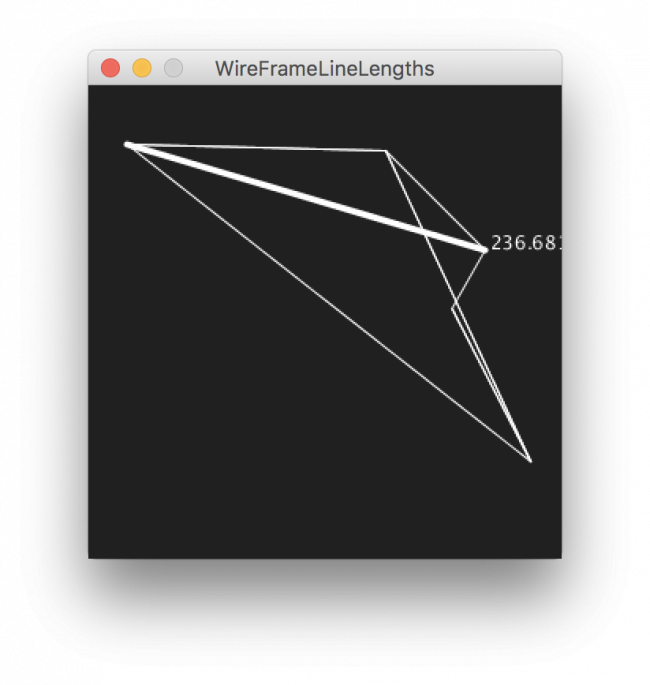#### Howdy, Stranger!

We are about to switch to a new forum software. Until then we have removed the registration on this forum.

# How to check whether mouse pointer is currently on the line drawn inside Canvas or not?

edited April 2017

Hi,

I am doing one visualization task, where I am drawing graph inside canvas through connecting edges as line function. Now I want to check whether my mouse is over the line or not. If it is then I would like to show the distance between this two vertices. Can anyone tell me here how can I do it with P5.js ?

Tagged:

• edited April 2017 Answer ✓
• If you have a list of points, then you need to have them sort. The task is as easy as checking the two points in the array that the mouse straddles.

Kf

• @GoToLoop The issue is I have an array of lines. Can I check parallelly on all lines that whether my mouse pointer is on them or not? Is p5 so fast to check on all lines?

• Dunno. Are all of those lines form a polygon area? :-/
http://www.JeffreyThompson.org/collision-detection/poly-point.php

If they're random lines everywhere, you've gotta iterate over them 1 by 1.
Once found a match, quit the loop prematurely. That's all I know. ^#(^

•They are lines of a connected graph. Actually, I want to show the distance of this line which I have already calculated and having an each edge as an object.

• Sorry, not good at all on 3D here. :(

• Is this a 3D model or are you working in 2D?

If it is 3D, you can project all points to a common plane. Then you need to find the point closest to your mouse pointer. You will then get all the lines associated to this point and you should find which line is closer to your mouse pointer. When you find that line, you can get the second point associated to that line.

If this is a 3D problem, there is a small issue if you change the perspective of your camera. There are perspectives where, for example, two points will overlap. Then the algorithm above will only detect one point.

Now, if this is a 2D, then GoToLoop's link is the best bet. You test your mouse position against all lines and find the one the mouse pointer is close to.

Kf

• Here is a related example in Processing (Java mode) rather than p5.js.

`lines` is an array of `PShape` with vertices -- but it could all be done with a custom line class instead. It can't be easily done with an array of PShapes using LINE because then there is no access to the vertex data after the shape has been created.

``````// forum.processing.org/two/discussion/22122/how-to-check-whether-mouse-pointer-is-currently-on-the-line-drawn-inside-canvas-or-not
ArrayList<PShape> lines;

void setup() {
size(300, 300);
stroke(255);
frameRate(10);
lines = lineSet(6, 12);
}

void draw() {
background(32);
for (PShape s : lines) {
s.beginShape();
if (mouseLine(s.getVertex(0), s.getVertex(1))) {
s.strokeWeight(4);
text(s.getVertex(0).dist(s.getVertex(1)), s.getVertex(0).x, s.getVertex(0).y);
} else {
s.strokeWeight(1);
}
s.endShape();
shape(s, 0, 0);
}
}

void keyPressed() {
lines = lineSet(6, 12);
}

ArrayList<PShape> lineSet(int pointCount, int lineCount) {
ArrayList<PVector> points = new ArrayList<PVector>();
for (int i=0; i<pointCount; i++) {
}
PShape s;
ArrayList<PShape> lines = new ArrayList<PShape>();
for (int i=0; i<lineCount; i++) {
s = createShape();
s.beginShape();
PVector a = points.get((int)random(0, points.size()-1));
PVector b = points.get((int)random(0, points.size()-1));
s.vertex(a.x, a.y);
s.vertex(b.x, b.y);
s.endShape();
}
return lines;
}

boolean mouseLine(PVector a, PVector b) {
return linePoint(a.x, a.y, b.x, b.y, mouseX, mouseY);
}

// www.jeffreythompson.org/collision-detection/line-point.php
boolean linePoint(float x1, float y1, float x2, float y2, float px, float py) {
float d1 = dist(px, py, x1, y1);
float d2 = dist(px, py, x2, y2);
float lineLen = dist(x1, y1, x2, y2);
float buffer = 0.2;    // higher # = less accurate
if (d1+d2 >= lineLen-buffer && d1+d2 <= lineLen+buffer) {
return true;
}
return false;
}
``````